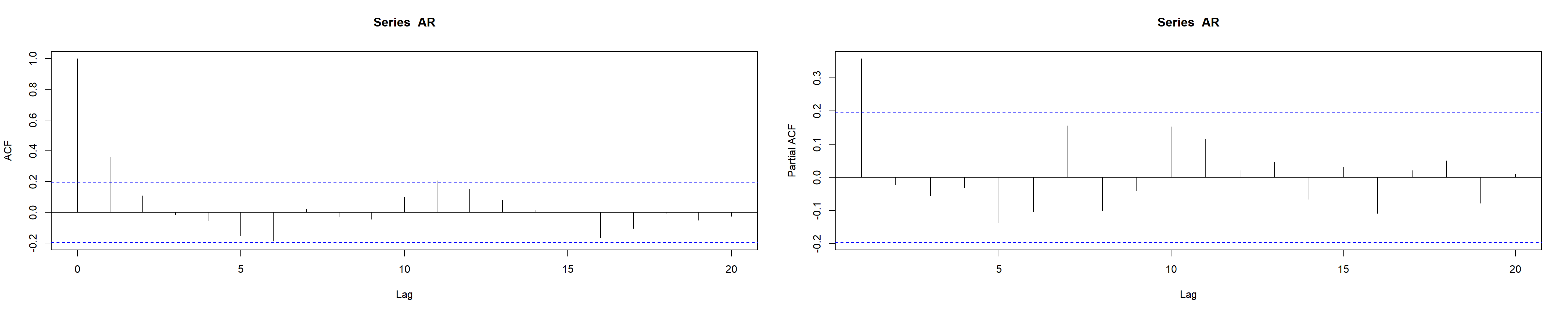### READING ACF AND PACF PLOTS:

From this youtube post. Also, here is a more extensive document with simulations found online.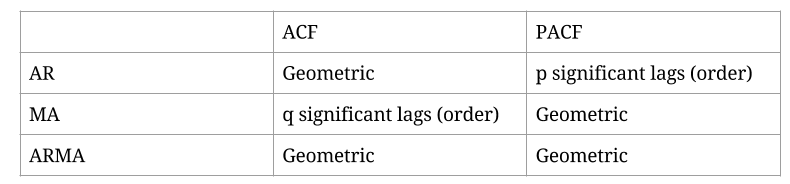Examples: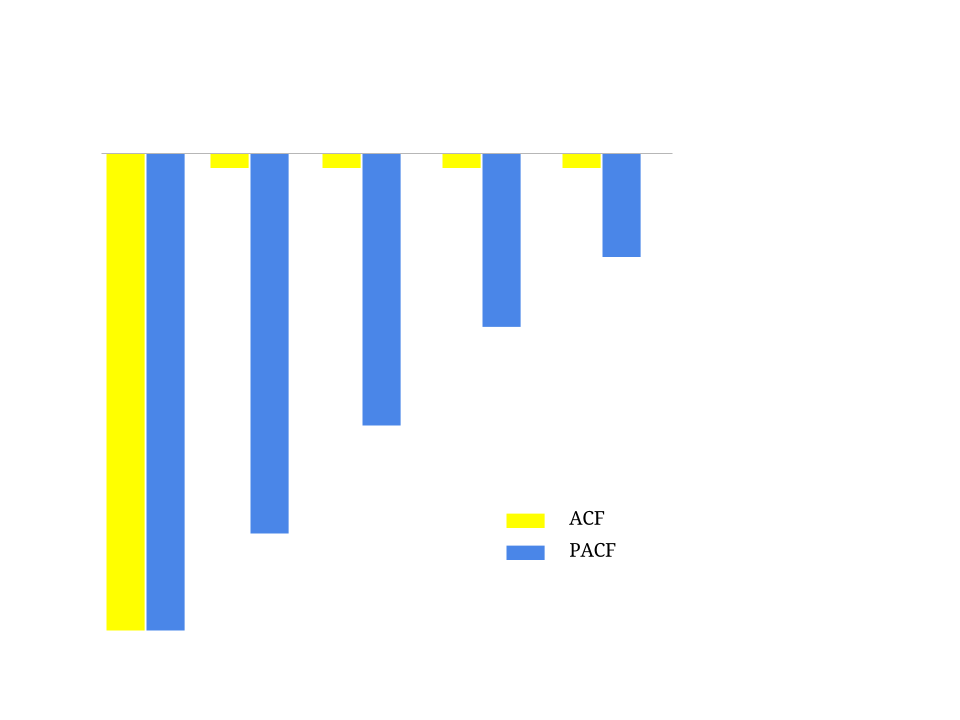On this plot the ACF is significant only once (in reality the first entry in the ACF is always significant, since there is no lag in the first entry - it’s the correlation with itself), while the PACF is geometric. Hence it is an MA(1) process.

The negative values in the plot respond to a process of the form $$\large\color{blue} {y_t} = k - \color{red}{\theta}\,\epsilon_{t-1}+\epsilon_t.$$

Here is a simulation of an MA(1) process with $$\theta = - 0.7:$$

set.seed(2017)
MA = arima.sim(model=list(ma = - 0.7), n = 100)
par(mfrow = c(1,2)); acf(MA); pacf(MA)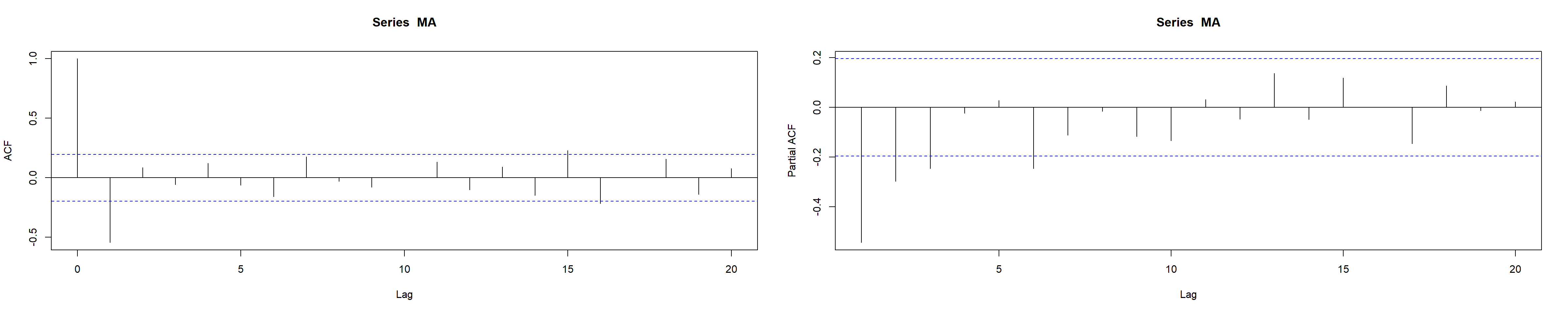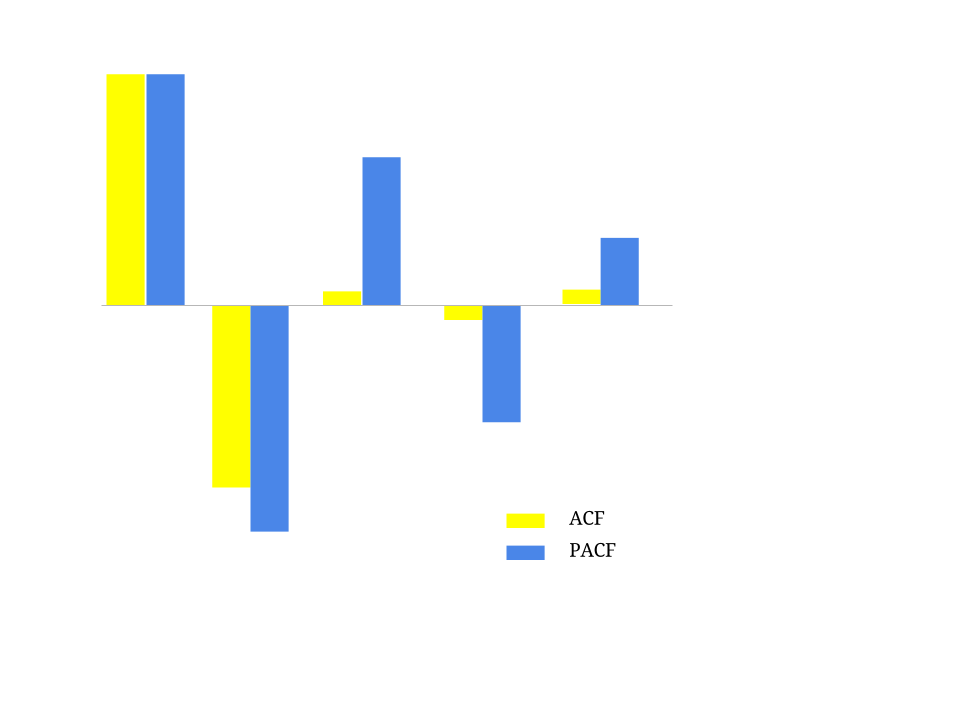In this example the ACF is significant in the first and second lags, while the PACF follows a geometric decay. It is again a MA process, but this time an MA(2) of the form: $$\large\color{blue} {y_t} = k+ \color{red}{\theta_1}\,\epsilon_{t-1}-\color{red}{\theta_2}\,\epsilon_{t-2}+\epsilon_t.$$

Here’s an R simulation with $$\theta_1 = 0.9$$ and $$\theta_2= -0.2:$$

set.seed(2017)
MA2 = arima.sim(list(ma= c(0.9, - 0.2)), n = 100)
par(mfrow = c(1,2));acf(MA2);pacf(MA2)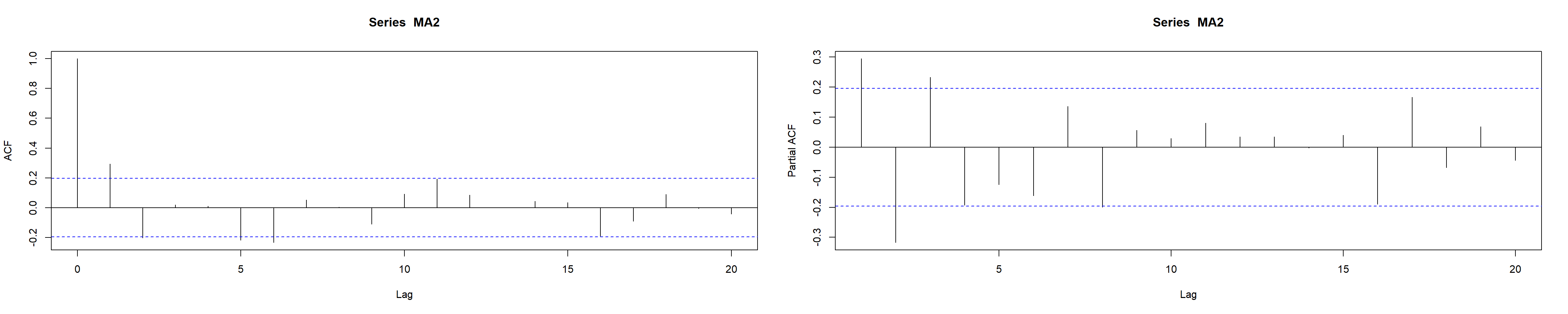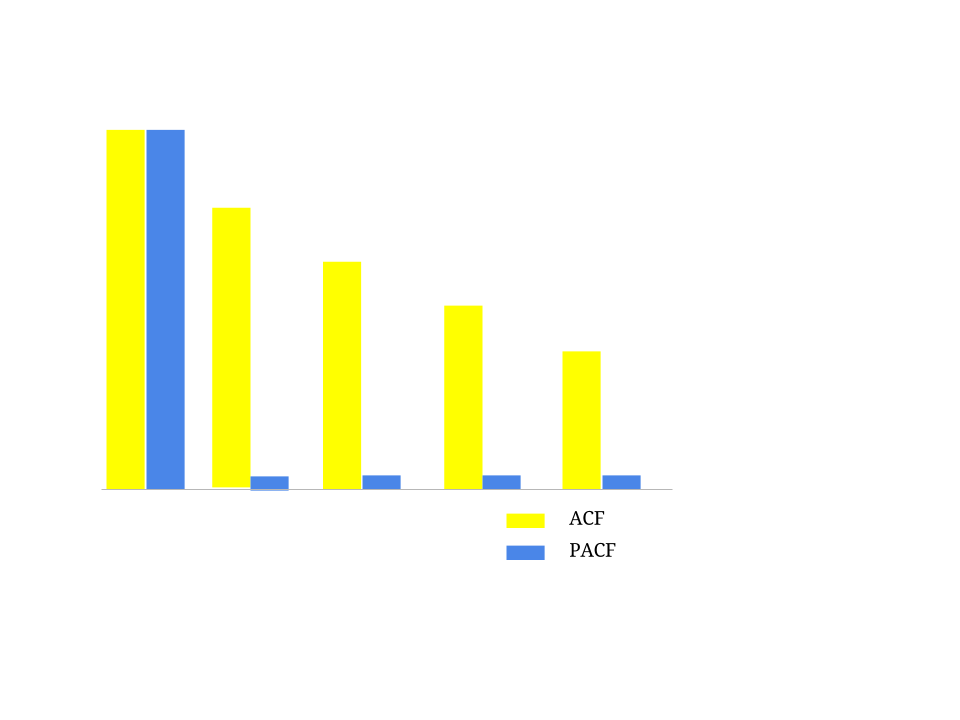Here the ACF decays geometrically, and the PACF shows only one significant lag. This is a AR(1) process of the form: $$\large \color{blue}{y_t} = c + \color{red}{\rho}\, \color{blue}{y_{t-1}}+\epsilon_t.$$

Here is the simulation in R with $$\rho = 0.9:$$

set.seed(2017)
AR = arima.sim(model=list(ar = .9), n = 100)
par(mfrow = c(1,2));acf(AR);pacf(AR)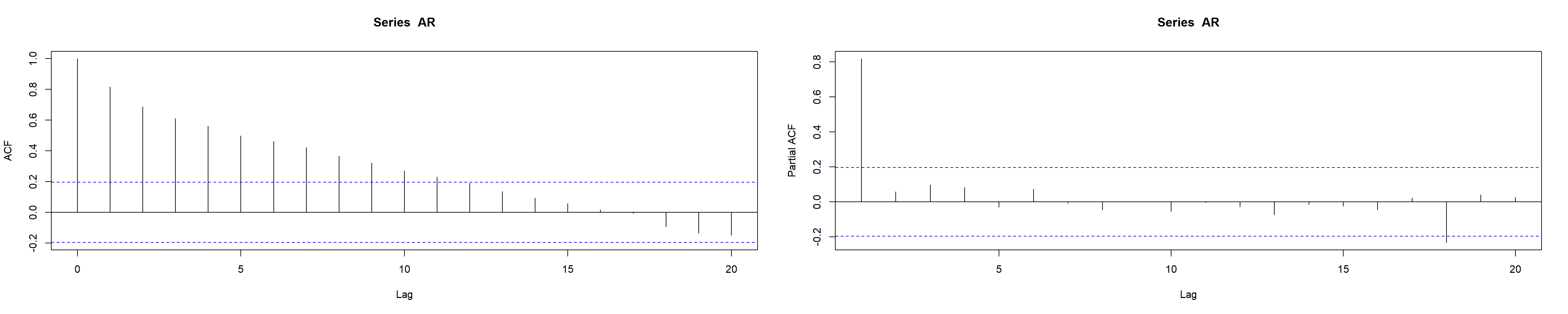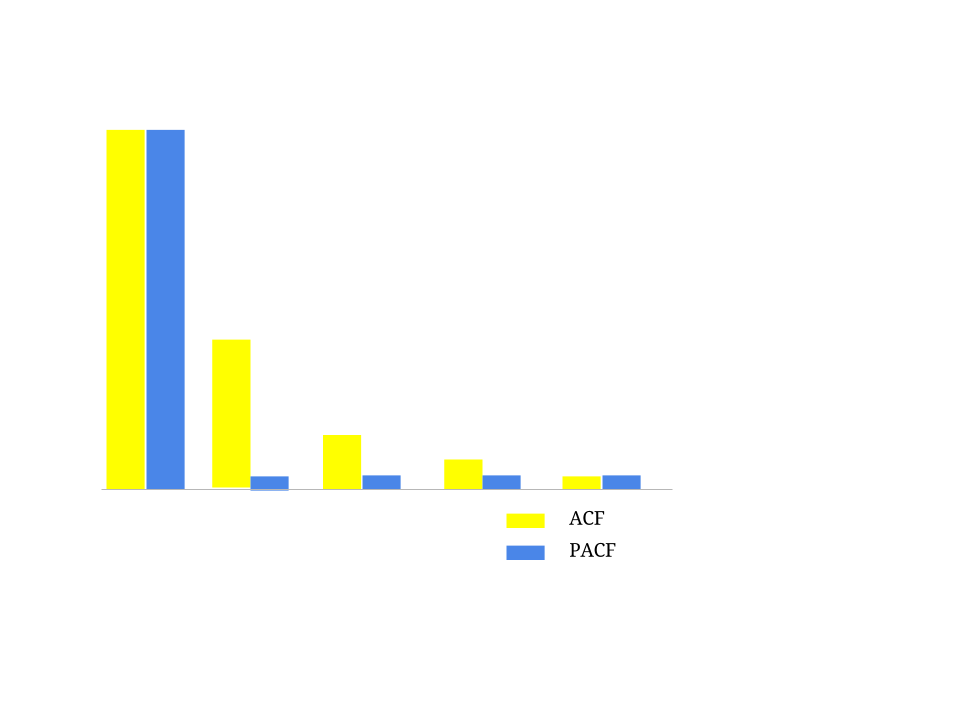This is again an AR(1) process, but with a faster decay, $$\rho=0.5:$$

set.seed(2017)
AR = arima.sim(model=list(ar = .5), n = 100)
par(mfrow = c(1,2));acf(AR);pacf(AR)